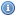# Calculus Books, Documents, and Lectures

 Title Year DescriptionAdvanced Calculus, Revised Edition 1990This book divides roughly into a first half which develops the calculus (principally the differential calculus) in the setting of normed vector spaces, and a second half which deals with the calculus of differentiable manifolds.Calculus 1991This book is well organized, covers single variable and multivariable calculus in depth, and is rich with applications.Calculus IA complete, concise, and well put together set of notes for a Calculus I course.Calculus IIA complete, concise, and well put together set of notes for a Calculus II course.Calculus IIIA complete, concise, and well put together set of notes for a Calculus III course.Calculus Without Limits 2005This text is a calculus primer. This book is shorter than the typical calculus book in that it only covers those ideas that are central to an understanding of the calculus of a real-valued function of a single real-variable.Calculus on the WebCalculus on the Web is an internet utility for learning and practicing calculus. The principal purpose is to provide the opportunity to learn and practice problems in calculus in a friendly environment via the internet.Differential Equations 2006This is a complete online course (MIT OpenCourseWare) on Differential Equations.First-Year Calculus 2006Lecture notes for a first year calculus course compiled over 25 years.Many Variable Advanced Calculus 2007This book is directed to people who have a good understanding of the concepts of one variable calculus including the notions of limit of a sequence and completeness of R. It develops multivariable advanced calculus.Multivariable Calculus 1997This is a textbook for a course in multivariable calculus. It has been used for the past few years at Georgia Tech.Notes on Differential Equations 2005These are introductory notes on ordinary and partial differential equations. Assumed background is calculus and a little physics. Linear algebra is not assumed, and is introduced here in four of the lectures.One Variable Advanced Calculus 2007This is text places calculus as part of mathematics and involves proofs and definitions, not algorithms and busy work.Stochastic CalculusAn informal introduction to Stochastic Calculus, especially to the Ito integral and some of its applications.The Calculus Bible 2010A study aide for high school students who have completed the Advanced Placement AB Calculus curriculum.The Calculus Bible: The New Testament 2010A review guide to the AP BC Calculus examTopics in CalculusThese notes present some ideas which the author believes that almost everyone who uses the integral as a tool takes for granted, but which are often not taught systematically and which seem to be very difficult for many students to learn.Vector Calculus 2008This is a text on elementary multivariable calculus, designed for students who have completed courses in single-variable calculus.Visual Calculus 2001A collection of tutorials, interactive modules (LiveMath, Java, and Javascript) which can be used by either students or faculty and includes detailed instructions for TI-85 and TI-86 graphing calculators.Yet Another Calculus Text - A Short Introduction with InfinitesimalsAn introduction to calculus based on the hyperreal number system. Intended for readers who already have some familiarity with calculus.